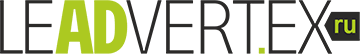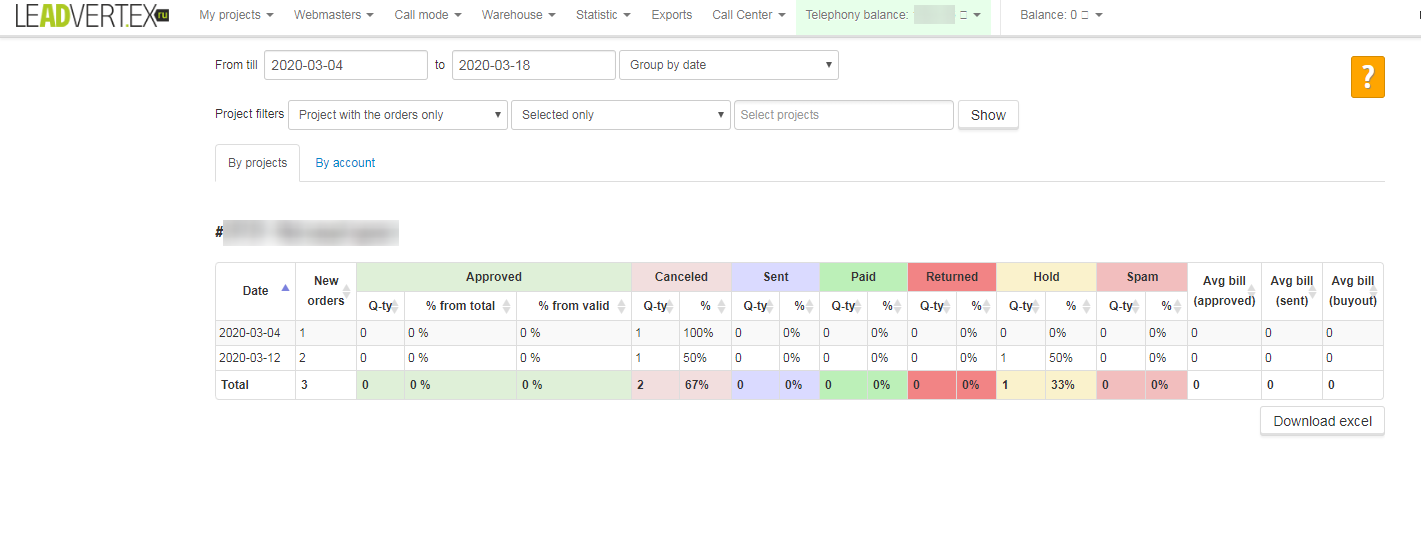### Conversions

Conversion statistics is in the project - Statistics - Conversion statistics. Statistics by conversions is shows how many orders are received every day and by what groups they are distributed. If the order arrived today, and it was confirmed the next day, then this confirmed order will be displayed in the statistics for the day when this order arrived in the admin panel.• The “Transitions” column shows how many transitions were on the landing page.
• The “Orders” column shows the number of orders for the current day and for the selected period.
• The “CR” column shows the ratio of orders to conversions as a percentage.
• The “Envelope” column shows the ratio of orders to transitions as a proportion.
• The “Return” column shows how many orders from new orders for the current period are moved to the statuses with the “Return” group
• The “Error / Spam / Double” column shows how many orders from new orders for the current period are moved to the statuses with the “Spam / Errors / Double” group
• The “Canceled” column shows how many orders from new orders for the current period are moved to statuses with the group “Canceled”
• The “Processing” column shows how many orders from new orders for the current period remain in statuses with the “Processing” group
• The “Accepted” column shows how many orders from new orders for the current period are moved to statuses with the “Accepted” group
• The “Paid” column shows how many orders from new orders for the current period are moved to the statuses with the group “Paid”
• The tab “Personal, General” at the top divides the statistics into only admin orders and all orders together with webmasters.

### Account statistics

• New orders - shows the number of orders received on the current day
• Accepted - how many orders are accepted from those received in the current period
• Approve of the total number - the percentage of orders received of the total number received in the current period
• Approve from valid orders - percentage of accepted orders from valid orders received in the current period
• Canceled - shows the number of canceled orders among received on the current day
• Remained in processing - how many orders remained in the status “Processing”
• Spam - how much has been moved to the "Spam" status
• Average bill - the average cost of accepted orders

## Call back application

We work from 8 am till 8 pm (moscow time) every day.
Please state convenient time for calling back at the working hours of our technical support service!